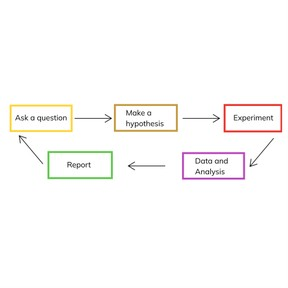The scientific method

# The scientific method

I can define and apply the steps of the scientific method.

No account needed.8,000 schools use Gynzy92,000 teachers use Gynzy1,600,000 students use Gynzy

## General

The scientific method is a set of steps that scientists can use to investigate and solve problems. The scientific method is not always linear and sometimes the order or name of steps varies slightly, but one thing that is constant is that the scientific method is used to test a hypothesis that can be tested and observed.

## Learning objective

This lesson will teach students to define and apply the steps of the scientific method.

## Introduction

Students are presented with a hypothetical situation where their teacher wants to buy them candy, but is afraid that this will make them unable to focus on their work. They are asked to talk with a partner about how this situation could be tested.

## Instruction

Here are the basic steps of the scientific method:

- Ask a question or imagine a hypothetical scenario
- Make a hypothesis, or a guess or theory, about the answer or solution to the problem or question posed
- Experiment so that you can see how the scenario plays out and if your hypothesis was correct
- Collect and analyze data from your experiment to determine the outcome
- Repeat if necessary

Remember that the hypothesis you propose doesn't need to be correct. Scientists make mistakes all the time! The important thing is that you are trying to solve a testable problem and analyze your findings to understand if you have solved the problem or not.

## Closing

Imagine a student in your class proposes this hypothesis: if a star and a planet collide, they will both explode. Discuss why or why not this is a good hypothesis to test through the scientific method.

### The online teaching platform for interactive whiteboards and displays in schools

• Save time building lessons

• Manage the classroom more efficiently

• Increase student engagement